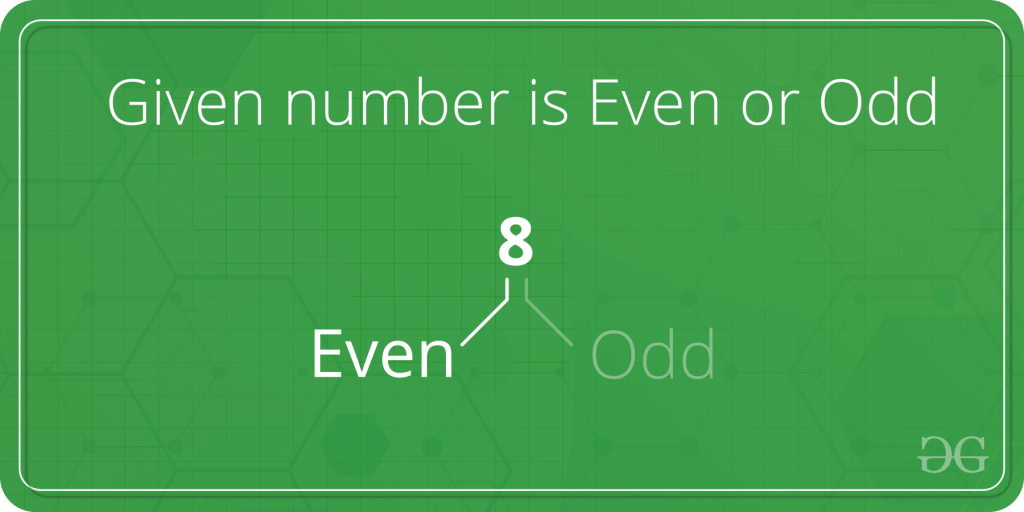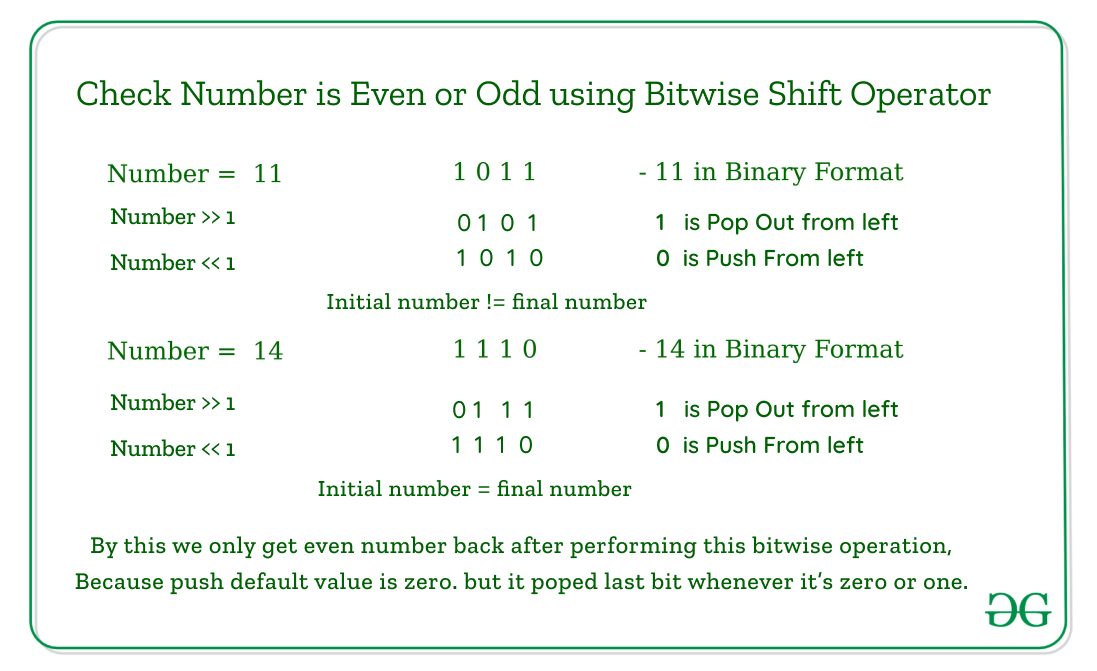Open In App

# Check whether a given number is even or odd

Given a number, check whether it is even or odd.Examples :

```Input: 2
Output: even

Input: 5
Output: odd```
Recommended Practice

One simple solution is to find the remainder after dividing by 2.

Method 1:

## C++

 `// A simple C++ program to``// check for even or odd``#include ``using` `namespace` `std;`` ` `// Returns true if n is``// even, else odd``bool` `isEven(``int` `n) { ``return` `(n % 2 == 0); }`` ` `// Driver code``int` `main()``{``    ``int` `n = 101;``    ``isEven(n) ? cout << ``"Even"` `: cout << ``"Odd"``;`` ` `    ``return` `0;``}`

## Java

 `// Java program  to``// check for even or odd`` ` `class` `GFG {``    ``// Returns true if n is even, else odd``    ``public` `static` `boolean` `isEven(``int` `n)``    ``{``        ``return` `(n % ``2` `== ``0``);``    ``}`` ` `    ``// Driver code``    ``public` `static` `void` `main(String[] args)``    ``{``        ``int` `n = ``101``;``        ``if` `(isEven(n) == ``true``)``            ``System.out.print(``"Even"``);``        ``else``            ``System.out.print(``"Odd"``);``    ``}``}`` ` `// This code is contributed by rishabh_jain`

## Python3

 `# A simple Python3 code``# to check for even or odd`` ` `# Returns true if n is even, else odd`` ` ` ` `def` `isEven(n):``    ``return` `(n ``%` `2` `=``=` `0``)`` ` ` ` `# Driver code``n ``=` `101``print``(``"Even"` `if` `isEven(n) ``else` `"Odd"``)`` ` `# This code is contributed by "Sharad_Bhardwaj".`

## C#

 `// C# program to``// check for even or odd``using` `System;`` ` `class` `GFG {``    ``// Returns true if n is even, else odd``    ``public` `static` `bool` `isEven(``int` `n)``    ``{``        ``return` `(n % 2 == 0);``    ``}`` ` `    ``// Driver code``    ``public` `static` `void` `Main()``    ``{``        ``int` `n = 101;``        ``if` `(isEven(n) == ``true``)``            ``Console.WriteLine(``"Even"``);``        ``else``            ``Console.WriteLine(``"Odd"``);``    ``}``}`` ` `// This code is contributed by vt_m`

## PHP

 ``

## Javascript

 ``

Output

`Odd`

Time Complexity: O(1)
Auxiliary Space: O(1)

Method 2 :

A better solution is to use bitwise operators. We need to check whether the last bit is 1 or not. If the last bit is 1 then the number is odd, otherwise always even.

Explanation:

``` input : 5    // odd
00000101
& 00000001
--------------
00000001
--------------

input : 8     //even
00001000
& 00000001
--------------
00000000
--------------```

Below is the implementation of the idea.

## C++

 `// A simple C++ program to``// check for even or odd``#include ``using` `namespace` `std;`` ` `// Returns true if n is``// even, else odd``bool` `isEven(``int` `n)``{`` ` `    ``// n & 1 is 1, then``    ``// odd, else even``    ``return` `(!(n & 1));``}`` ` `// Driver code``int` `main()``{``    ``int` `n = 101;``    ``isEven(n) ? cout << ``"Even"` `: cout << ``"Odd"``;`` ` `    ``return` `0;``}`

## C

 `#include ``#include `` ` `int` `main()``{``    ``int` `n = 101;``    ``if` `(n % 2 == 0) {``        ``printf``(``"Even"``);``    ``}``    ``else` `{``        ``printf``(``"Odd"``);``    ``}``    ``return` `0;``}`

## Java

 `// Java program to``// check for even or odd`` ` `class` `GFG {``    ``// Returns true if n``    ``// is even, else odd``    ``public` `static` `boolean` `isEven(``int` `n)``    ``{``        ``if` `((n & ``1``) == ``0``)``            ``return` `true``;``        ``else``            ``return` `false``;``    ``}`` ` `    ``// Driver code``    ``public` `static` `void` `main(String[] args)``    ``{``        ``int` `n = ``101``;``        ``if` `(isEven(n) == ``true``)``            ``System.out.print(``"Even"``);``        ``else``            ``System.out.print(``"Odd"``);``    ``}``}`` ` `// This code is contributed by rishabh_jain`

## Python3

 `# A Python3 code program``# to check for even or odd`` ` `# Returns true if n is even, else odd`` ` ` ` `def` `isEven(n):`` ` `    ``# n&1 is 1, then odd, else even``    ``return` `(``not``(n & ``1``))`` ` ` ` `# Driver code``n ``=` `101``print``(``"Even"` `if` `isEven(n) ``else` `"Odd"``)`` ` `# This code is contributed by "Sharad_Bhardwaj".`

## C#

 `// C# program  to``// check for even or odd``using` `System;`` ` `class` `GFG {``    ``// Returns true if n``    ``// is even, else odd``    ``public` `static` `bool` `isEven(``int` `n)``    ``{``        ``if` `((n & 1) == 0)``            ``return` `true``;``        ``else``            ``return` `false``;``    ``}`` ` `    ``// Driver code``    ``public` `static` `void` `Main()``    ``{``        ``int` `n = 101;``        ``if` `(isEven(n) == ``true``)``            ``Console.WriteLine(``"Even"``);``        ``else``            ``Console.WriteLine(``"Odd"``);``    ``}``}`` ` `// This code is contributed by vt_m.`

## PHP

 ``

## Javascript

 ``

Output

`Odd`

Time Complexity: O(1)
Auxiliary Space: O(1)

Method 3: Another approach is by using bitwise left-shift and right-shift operators. The logic behind this implementation is about regenerating the value after the right shift and left shift. We all know even numbers have zero as the last bit and odd have one as the last bit. When we bitwise right shift any number then the last bit of the number piped out whenever it is even or odd. Next, we did a bitwise left shift, then our bit shifted by one. Now last bit placed is empty which is by default filled by a zero. During all these odd numbers changed their value but even remains the same. That’s how by comparing the initial and final value we decide number is even or odd.below is the implementation of the above logic.

## C++

 `#include ``using` `namespace` `std;`` ` `int` `main()``{`` ` `    ``int` `a = 4;``    ``// To take input from user, prefer below code``    ``// int a ;``    ``// cout<<"Enter an number: " <<"\n";``    ``// cin>>a;`` ` `    ``if` `(a == (a >> 1) << 1) {``        ``cout << ``"Number is Even: "` `<< a << endl;``    ``}``    ``else` `{``        ``cout << ``"Number is Odd: "` `<< a << endl;``    ``}`` ` `    ``// below is short hand``    ``// a==(a>>1)<<1? printf("Number %d is``    ``// even.\n",a):printf("Number %d is odd.\n",a);`` ` `    ``return` `0;``}`

## Java

 `// Java implementation of above approach``import` `java.io.*;``class` `HelloWorld {``    ``public` `static` `void` `main(String[] args)``    ``{``        ``int` `a = ``4``;`` ` `        ``// To take input from user, prefer below code``        ``// int a ;``        ``// printf("Enter an number: \n");``        ``// scanf("%d",&a);``        ``if` `(a == (a >> ``1``) << ``1``) {``            ``System.out.printf(``"Number %d is even.\n"``, a);``        ``}``        ``else` `{``            ``System.out.printf(``"Number %d is not even.\n"``,``                              ``a);``        ``}`` ` `        ``// below is short hand``        ``// a==(a>>1)<<1? printf("Number %d is``        ``// even.\n",a):printf("Number %d is odd.\n",a);``    ``}``}`` ` `// This code is contributed by karandeep1234.`

## Python3

 `a ``=` `4``if` `a ``=``=` `(a >> ``1``) << ``1``:``    ``print``(``"Number is Even:"``, a)``else``:``    ``print``(``"Number is Odd:"``, a)`

## C#

 `// C# implementation of above approach``using` `System;`` ` `class` `HelloWorld {``    ``public` `static` `void` `Main(``string``[] args)``    ``{``        ``int` `a = 4;`` ` `        ``if` `(a == (a >> 1) << 1) {``            ``Console.WriteLine(``"Number "` `+ a + ``" is even."``);``        ``}``        ``else` `{``            ``Console.WriteLine(``"Number "` `+ a``                              ``+ ``" is not even."``);``        ``}`` ` `        ``// below is short hand``        ``// a==(a>>1)<<1? printf("Number %d is``        ``// even.\n",a):printf("Number %d is odd.\n",a);``    ``}``}`` ` `// This code is contributed by karandeep1234.`

## Javascript

 `const a = 4;`` ` `// To take input from user, prefer below code``// let a = prompt("Enter a number: ");`` ` `// Check if the number is even``if` `(a == (a >> 1) << 1) {``    ``console.log(``"Number is Even: "` `+ a);``}``else` `{``    ``console.log(``"Number is Odd: "` `+ a);``}`` ` `// This code is contributed by Shivam Tiwari`

Output

```Number 4 is even.
Number is Even: 4```

Time Complexity: O(1)
Auxiliary Space: O(1)

This article is contributed by Prabhat Raushan. If you like GeeksforGeeks and would like to contribute, you can also write an article using write.geeksforgeeks.org or mail your article to review-team@geeksforgeeks.org. See your article appearing on the GeeksforGeeks main page and help other Geeks.